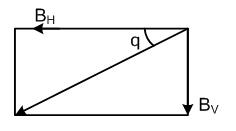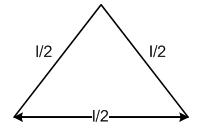×#### Thank you for registering.

One of our academic counsellors will contact you within 1 working day.

Click to Chat

1800-1023-196

+91-120-4616500

CART 0

• 0

MY CART (5)

Use Coupon: CART20 and get 20% off on all online Study Material

ITEM
DETAILS
MRP
DISCOUNT
FINAL PRICE
Total Price: Rs.

There are no items in this cart.
Continue Shopping• Complete Physics Course - Class 11
• OFFERED PRICE: Rs. 2,968
• View Details

```Solved Questions on Magnetics

Magnetics is a significant topic of IIT JEE Physics. It is important to attain expertise in this topic as it fetches quite a few questions in the JEE. It is vital to focus on areas like magnetic field, magnetic lines of force and magnetic flux as with a bit of effort, one can score a lot in these topics.

Now we discuss some questions on these topics. These topics have been discussed in detail in the last sections.

Q1. At any place on earth, the horizontal component of earth's magnetic field is √3 times the vertical component. The angle of dip at that place will be-

(A) 60o                  (B) 45o                  (C) 90o                  (D) 30oSol.

Since, it is given in the question that the horizontal component is √3 times the vertical component,
If horizontal component is assumed to be BH and the vertical component is assumed to be BY, then we get the relation, BH = √3BY.
Hence, tan Φ = Bv/BH = Bv /√3Bv = √3
So, Φ =30 o. Hence, (D) is the correct option.

Q2. The horizontal component of earth's magnetic field at any place is 0.36×10–4 Weber/m2. If the angle of dip at that place is 60o then the value of vertical component of earth's magnetic field will be-(in Wb/m2)-

(A) 0.12 × 10–4     (B) 0.24 × 10–4     (C) 0.40 × 10–4     (D) 0.62 × 10–4

Sol.

As we have done in the last problem,
BH tan Φ = 0.36 × 10–4 tan 60o
=  0.36 × 10–4 √3 = 0.62 × 10–4 Wb / m2
Hence, (D) is the correct option.

Q3. The value of angle of dip at a place on earth is 45o. If the horizontal component of earth's magnetic field is 5×10–5 Tesla then the total magnetic field of earth will be-

(A) 5/√2 × 10–5 Tesla                             (B) 10/√2 × 10–5 Tesla

(C) 15/√2 × 10–5 Tesla                           (D) zero

Sol.

Using the same formula again, we know

Total Magnetic Field B = √B2V + B2H = √B2H tan2 45o + B2H = BH√2

Hence, the answer is (A) 5√2 × 10–2 Wb / m2.

Q4. The ratio of intensities of magnetic field, at distances x and 2x from the centre of a magnet of length 2cm on its axis, will be-

(A) 4 : 1                 (B) 4 : 1 approx        (C) 8 : 1                (D) 8 : 1 approx

Sol.

Given that the length of the magnet is 2 cm.

We know that for finding the ratios of intensities of magnetic field

B = m0 / 4π 2M / (l3 + x3) >> m0 / 4π 2M / x3

Hence, the correct option is (A).

Q5. The length of a bar magnet is 10 cm and its pole strength is 10–3 Weber. It is placed in a magnetic field of induction 4π × 10–3 Tesla in a direction making an angle of 30o with the field direction. The value of torque acting on the magnet will be-

(A) 2π × 10–7 N-m                                (B) 2π × 10–5 N-m

(C) 0.5 × 102 N-m                                (D) 0.5N-m.

Sol.

The length of the bar magnet is 10cm.

Pole strength is given to be 10-3 Weber. The torque is given by

t = MB sin θ = m / B sin θ

= 10–3 × 0.1 4π × 10–3 × 0.5 = 2π × 10–7 N – m

Hence, the correct option is (A).

Q6.  A magnetic needle of magnetic moment 60 amp-m2 experiences a torque of 1.2 × 10–3 N-m directed in geographical north. If the horizontal intensity of earth's magnetic field at that place is 40 Wb/m2, then the angle of declination will be

(A) 30°                        (B) 45°                  (C) 60°                  (D) 90°

Sol.

The magnetic moment of the magnetic needle is given to be 60 amp-m2.

We know t = MB sin θ

So, sin θ = t / MB

= 1.2 × 10–3 / 60 × 40 × 10–6

= 1/2

Hence sin θ =1/2 , so θ = 30o.

Q7. The ratio of total intensities of magnetic field at the equator and the poles will be-

(A) 1 : 1                     (B)1 : 2                    (C) 2 : 1                 (D) 1 : 4

Sol.

Since we know that the total intensity of magnetic field remains constant, hence, Be/Bp = 1 : 1.

Q8. The magnetic flux density of magnetic field is 1.5 Wb/m2. A proton enters this field with a velocity of 2×107 m/s in a direction making an angle of 30o with the field. The force acting on the proton will be-

(A) 2.4 × 10–12 Newton                         (B) 0.24 × 10–12 Newton

(C) 24 × 10–12 Newton                          (D) 0.24 × 10–12 Newton

Sol.

The magnetic flux density is 1.5 Wb/m2.

The velocity of the proton is 2×107 m/s and makes an angle of 30o.

So, the force acting on the proton is given by

F = qvB sin θ = 1.6 × 10–19 × 2 × 107 × 1.5 × 0.5

= 2.4 × 10–12.

Hence, the correct answer is (A) 2.4 × 10–12 Newton.

Q9. The intensity of magnetic field at a point X on the axis of a small magnet is equal to the field intensity at another point Y on its equatorial axis. The ratio of distances of X and Y from the centre of the magnet will be-

(A) (B)–3                (B) (B)–3                     (C) 23                           (D) 21/3

Sol.

We are given a relation in the question that

The intensity of a magnetic field at a point X on the axis of a small magnet is equal to the field intensity at another point Y on its equatorial axis

Hence, m0 / 4π 2M / d3x = m0 / 4π M/d3y    so dx/dy = 21/3

The correct option is (D).

Q10. Two magnets A and B are equal in length, breadth and mass, but their magnetic moments are different. If the time period of B in a vibration magnetometer is twice that of A, then the ratio of magnetic moments will be-

(A)1/2                    (B) 2                      (C) 4                      (D) 12

Sol.

The question is demanding the relation between the time periods and magnetic moments. So we use the given formula

T = 2π √I / MB        M μ 1/T2

This gives (C) as the correct option.

Q11. The period of oscillation of a freely suspended bar magnet is 4 second. If it is cut into two equal parts lengthwise then the time period of each part will be-

(A) 4 sec.                 (B) 2 sec.                (C) 0.5 sec.            (D) 0.25 sec.

Sol.

The period of oscillation is 4 sec.

The time period is calculated with the help of the formula

T = 2π √I / MB = 2π √ml2/ 12 × m/b

= 4 sec.

Hence, the correct answer is (A).

Q12. The time period of a small magnet in a horizontal plane is T. Another magnet B oscillates at the same place in a similar manner. The size of two magnets is the same but the magnetic moment of B is four times that of A. The time period of B will be-

(A) T/4                    (B) T/2                    (C) 2T                    (D) 4T

Sol.

The time period in horizontal plane is T.

Let MB be the magnetic moment of B and MA be the magnetic moment of A.

Then, according to question, MB = 4MA.

Henec, using the formula, √MA/MB = TB/TA

or   TB = T × √MA/4MA = T/2

Hence, the correct option is (B).

Q13. The value of current enclosed by a circular path of radius 0.30 cm is 9.42 ampere. The value of magnetic field along the path will be-

(A) 500 amp/m                                     (B) 1000 Amp/m

(C) 5 × 104 Amp/m                               (D) Zero

Sol.

The value of current is 9.42 ampere.

The relation between current and magnetic field id given by the formula

H = i / 2pr = 9.42 / 2 × 314 × 3 × 10–3

= 500 amp/m, which gives (A) as the correct option.

Q14. A magnetic wire is bent at its midpoint at an angle of 60o. If the length of the wire is 1 and its magnetic moment is M then the magnetic moment of new shape of wire will be-

(A) 2 M                  (B) M                     (C) M/2                   (D) M/√2

Sol.The length of the wire is 1 and is bent at its midpoint. We get the shape as shown in the figure.

If M is the original magnetic moment, then the new magnetic moment is given by M' = M × 1/2 = M/2.

Q15. A current of 2 ampere is flowing in a coil of radius 50 cm and number of turns 20. The magnetic moment of the coil will be-

(A) 3.14 amp-m2                               (B) 31.4 amp-m2

(C) 314 amp-m2                                (D) 0.314amp-m2

Sol.

(B) M = μ R2Ni = 3.14 × 0.25 × 20 × 2 = 3.14 amp / m2.

Q16. 1000 turns per meter are wound over a Rowland ring of ferromagnetic material. On passing a current of 2 ampere in the coil, a magnetic field of 10 Wb/m2 is produced in it. The magnetizing force generated in the material will be-

(A) 1.2 × 10–3 A/m                        (B) 2.6 × 10–3 A/m

(C) 0.6 × 10–4 A/m                        (D) 2 × 10–3 A/m

Sol.

The current is of 2 ampere and the magnetic field is of 10 Wb/m2.

The relation between current, magnetic field and magnetic force is given by the formula

H = Ni / 2μr

= ni = 103 × 2

= 2 × 10-3 amp /m

Hence, magnetizing force is 2 × 10-3 amp /m.

Q17. A magnet makes 10 oscillations per minute at a place where the horizontal component of earth's magnetic field (H) is 0.33 oersted. The time period of the magnet at a place where the value of H is 0.62 oersted will be-

(A) 4.38 S             (B) 0.38 S             (C) 2.38 S             (D) 8.38 S

Sol.

The horizontal component of Earth’s surface is 0.33 oersted.

If the value of H is 0.62, then the time period is given by the relation

T μ 1/√H

So, T2 – T1 √H1 / H2 = 6 × √0.33/0.62

= 4.38 s.

Hence, this gives (A) as the correct option.

Q18. The magnetic induction inside a solenoid is 6.5×10–4T. When it is filled with iron medium then the induction becomes 1.4T. The relative permeability of iron will be-

(A) 1578               (B) 2355               (C) 1836               (D) 2154

Sol.

The given induction inside the solenoid is 6.5×10–4T.

It changes to 1.4T when filled with iron medium. Hence, the relative permeability is given by the relation

μ1 = B/B0 = 1.4 / 6.5 × 10–4

= 2154.

So, the correct option is (D).

Related Resources

Look into the Previous Papers with Solutions to get a hint of the kinds of questions asked in the exam.

You can get the knowledge of Recommended Books of Physics here.

```### Course Features

• 101 Video Lectures
• Revision Notes
• Previous Year Papers
• Mind Map
• Study Planner
• NCERT Solutions
• Discussion Forum
• Test paper with Video Solution